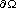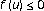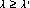# Existence results for classes of-Laplacian semipositone equations

## Abstract

We study positivesolutions to classes of boundary value problems of the forminon, wheredenotes the-Laplacian operator defined by;,is a parameter,is a bounded domain in;withof classand connected (if, we assume thatis a bounded open interval), andfor some(semipositone problems). In particular, we first study the case whenwhereis a parameter andis afunction such that,forandfor. We establish positive constantsandsuch that the above equation has a positive solution whenand. Next we study the case when(logistic equation with constant yield harvesting) whereandis afunction that is allowed to be negative near the boundary of. Hereis afunction satisfyingfor,, and. We establish a positive constantsuch that the above equation has a positive solution whenOur proofs are based on subsuper solution techniques.

## References

1. Berestycki H, Caffarelli LA, Nirenberg L: Further qualitative properties for elliptic equations in unbounded domains. Annali della Scuola Normale Superiore di Pisa. Classe di Scienze. Serie IV 1997,25(1–2):69–94. dedicated to E. De Giorgi

2. Brown KJ, Shivaji R: Simple proofs of some results in perturbed bifurcation theory. Proceedings of the Royal Society of Edinburgh. Section A. Mathematics 1982,93(1–2):71–82. 10.1017/S030821050003167X

3. Castro A, Maya C, Shivaji R: Nonlinear eigenvalue problems with semipositone structure. In Proceedings of the Conference on Nonlinear Differential Equations (Coral Gables, Fla, 1999), 2000, Texas, Electron. J. Differ. Equ. Conf.. Volume 5. Southwest Texas State University; 33–49.

4. Chhetri M, Oruganti S, Shivaji R: Positive solutions for classes of-Laplacian equations. Differential and Integral Equations 2003,16(6):757–768.

5. Clément Ph, Peletier LA: An anti-maximum principle for second-order elliptic operators. Journal of Differential Equations 1979,34(2):218–229. 10.1016/0022-0396(79)90006-8

6. Clément Ph, Sweers G: Existence and multiplicity results for a semilinear elliptic eigenvalue problem. Annali della Scuola Normale Superiore di Pisa. Classe di Scienze. Serie IV 1987,14(1):97–121.

7. Drábek P, Hernández J: Existence and uniqueness of positive solutions for some quasilinear elliptic problems. Nonlinear Analysis 2001,44(2):189–204. 10.1016/S0362-546X(99)00258-8

8. Drábek P, Krejčí P, Takáč P: Nonlinear Differential Equations, Chapman & Hall/CRC Research Notes in Mathematics. Volume 404. Chapman & Hall/CRC, Florida; 1999:vi+196.

9. Fleckinger-Pellé J, Takáč P: Uniqueness of positive solutions for nonlinear cooperative systems with the-Laplacian. Indiana University Mathematics Journal 1994,43(4):1227–1253. 10.1512/iumj.1994.43.43053

10. Hai DD: On a class of sublinear quasilinear elliptic problems. Proceedings of the American Mathematical Society 2003,131(8):2409–2414. 10.1090/S0002-9939-03-06874-6

11. Hai DD, Shivaji R: Existence and uniqueness for a class of quasilinear elliptic boundary value problems. Journal of Differential Equations 2003,193(2):500–510. 10.1016/S0022-0396(03)00028-7

12. Oruganti S, Shi J, Shivaji R: Diffusive logistic equation with constant yield harvesting. I. Steady states. Transactions of the American Mathematical Society 2002,354(9):3601–3619. 10.1090/S0002-9947-02-03005-2

13. Oruganti S, Shi J, Shivaji R: Logistic equation wtih the-Laplacian and constant yield harvesting. Abstract and Applied Analysis 2004,2004(9):723–727. 10.1155/S1085337504311097

## Author information

Authors

### Corresponding author

Correspondence to R Shivaji.

## Rights and permissions

Reprints and Permissions

Oruganti, S., Shivaji, R. Existence results for classes of-Laplacian semipositone equations. Bound Value Probl 2006, 87483 (2006). https://doi.org/10.1155/BVP/2006/87483

• Accepted:

• Published:

• DOI: https://doi.org/10.1155/BVP/2006/87483

### Keywords

• Differential Equation
• Partial Differential Equation
• Ordinary Differential Equation
• Positive Constant
• Functional Equation